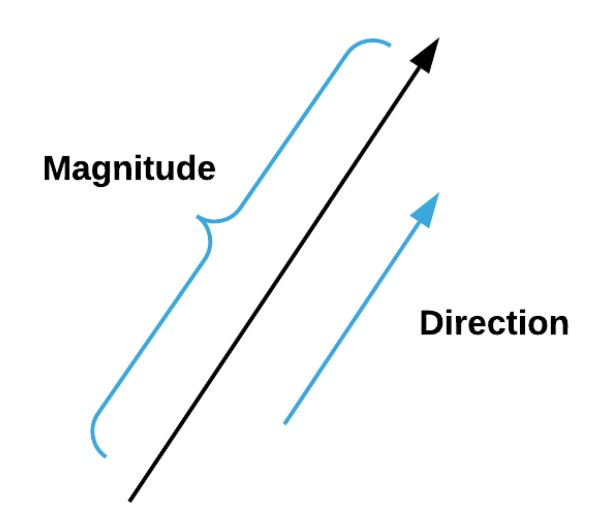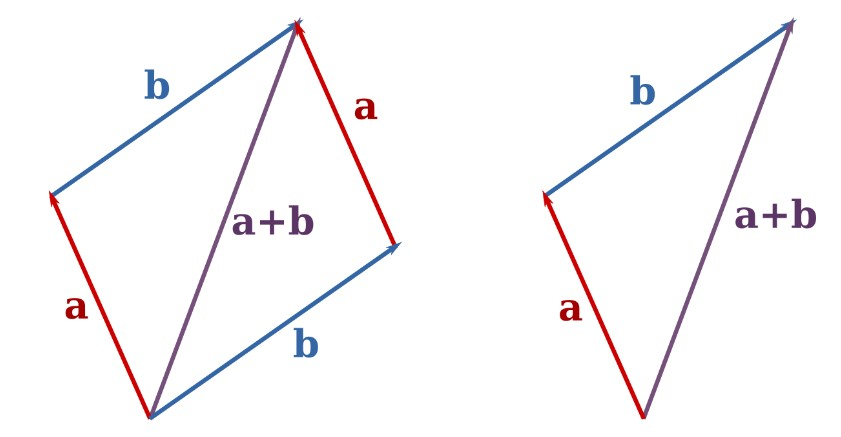Input the values of coefficients of the unit vectors in the vector addition calculator.

(Ai → Aj → Ak) + (Bi → Bj → Bk)
i
j
k
i
j
k

Give Us Feedback

Vector addition calculator is used to add vectors that exist in 2 or 3 dimensions. This vector sum calculator adds 2d vectors as well as 3d vectors.

## What is a vector?

According to Wikipedia:

“In mathematics and physics, a vector is an element of a vector space.”

It is such an element that has both a magnitude number and a direction. They exist in the cartesian plane. Vectors have sub vectors, namely unit vectors, to represent the direction.Vector addition is a simple process. The only restriction is that only identical unit vectors can be added. Adding vectors can be elaborated through an example.Example:

Add the vector A and B, where;

A = 3i + 2j + 3k

B =   i + 3j + 0k

Solution:

i          j          k

3          2         3

+     1          3         0

_________________

4          5         3

Vector subtraction is same as vector addition. The only difference is; we subtract the numbers instead of adding them. Using a vector subtraction calculator can save you a lot of time.

### Math Tools